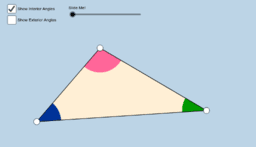•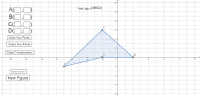### Geometry Transformations - Draw the Answer

Activity

•### Systems of Equations Practice

Activity

•### Basic Systems of Equations

Activity

•### Tangent and Normal Line Practice

Activity

•### Factoring Practice

Activity

•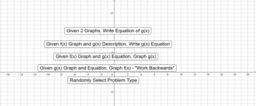### Function Transformations with Horizontal Stretch

Activity

•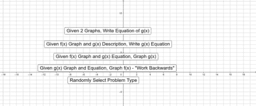### Function Transformations

Activity

•### Limit Practice

Activity

•### Graphing Lines

Activity

•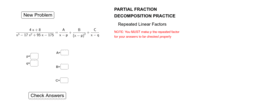### Partial Fraction Decomposition - Repeated Linear Factors

Activity

•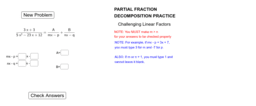### Partial Fraction Decomposition - Challenging Linear Factors

Activity

•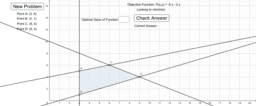### Linear Programming

Activity

•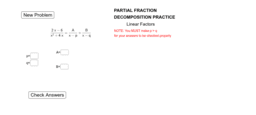### Partial Fraction Decomposition - Linear Factors

Activity

•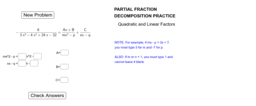### Partial Fraction Decomposition - Quadratic & Linear Factors

Activity

•### Writing Equations of Lines

Activity

•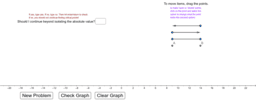### Absolute Value Equations and Inequalities

Activity

•### Absolute Value Equations and Inequalities - Geometric

Activity

•### Systems with Variables in Denominator

Activity

•### Map of Indiana

Activity

•